# Methods of Expressing the Concentration of a Solution

Methods of Expressing the Concentration of a Solution

The concentration of a solution can be expresses in a number of ways. The important methods are:

1. ## Mass Volume Percentage (W/V)

It is s defined as the mass of solute present in 100 mL of solution.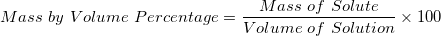1. ## Mole Fraction

It is defined as the ratio of the number of moles of that component to the total number of moles of all components.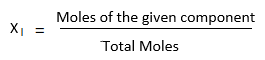In a binary solution for 2 components, A and B.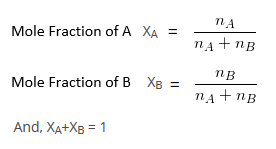1. ## Molarity (M)

Molarity of a solution is the number of gram molecules of the solute present in one litre of the solution.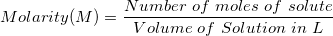Units of molarity: mol L-1

1. ## Molality (m)

It is defined as the number of moles of solute dissolved in 1 Kg of the solvent.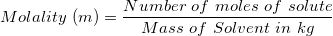Units of molarity: mol kg-1

##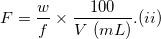Where,

• w = weight of solute,

• f = formula weight of solute

• V= volume of solution

• nf = no. of gram formula weight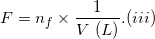1. ## Formality (F)

It is defined as the number of gram equivalents of the solute present in one litre of the solution.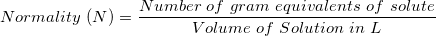1. ## Parts per million

It is defined as the Number of the parts of components by Total no of parts of all components of the solution per million.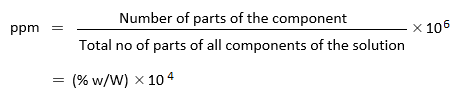Post By : Divya SIngh 25 Dec, 2018 3275 views Chemistry